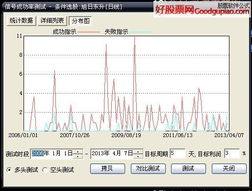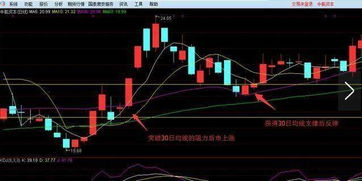# 准确率超高的短线选股公式,底部突破涨停板选股公式

MACD四大经典买入信号：

1、漫步青云。DIF线在零轴上死叉DEA线，继续下穿零轴，在零轴或零轴以上金叉DEA。此时的K线形态往往是穿越了、或正在穿越重要的均线。该形态形成是股价在探底回升途中做盘整，也有的是筑底形态，呈上攻之势，应理解为积极介入信号，应果断入市。2、海底电缆。MACD在零轴下运行一个月以上的较长时间，DIF在零轴下金叉DEA后，两线粘合成一线、数值几乎相等，两线一旦开始向上多头发散，就可考虑 买入。0轴以下的海底电缆形态的形成，多为股价在下跌探底以后，抛盘穷尽时呈现的底部形态，这时主力介入，进入压箱底吸货所致，应理解为择机入市。3、空中缆车。DIFF线在0轴之上死叉DEA线，但不下穿0轴，过几天即再次在0轴以上金叉DEA。该形态的出现多为上档盘整，主力洗盘所为，股价做短暂的调整后，呈现强劲上升动力，可理解为积极介入信号，可果断买入，如能连续放量更可坚决看多。4、天鹅展翅。DIFF在0轴以下金叉DEA线，随后没有上穿0轴就回调，向DEA靠拢，MACD红柱缩短，但没有死叉DEA就再次反转向上，同时配合 MACD红柱加长，便形成天鹅展翅形态。该形态的形成多为底部形态，是股价在下跌探底之后，抛盘穷尽之时呈现的底部形态，应理解为主力建仓区域，可择机介入。（图1） C点处,出现MACD金叉,但是零轴线之下的金叉。按照广通技术讲,零下金叉都是弱市中的反弹买进点,买入后能涨多少就很难说了,多数是亏损的。因此,广通有句话叫“水下金叉易受潮”,就是劝你少买水下金叉。有人问,在MACD走到“零上红”色区间前,总有最后一个“水下金叉”,买进不就买在底部吗？是的,但广通技术不追求最后一个水下金叉这种底部。

“MACD零下做空,零上做多,零上红金叉坚决做多,二次零上红金叉更坚决做多”,这是广通纪律。R点后,股价进入2 3区间,MACD进入零上红区间,这时可以买股票了。然而,像 600051航天晨光这样的个股,买进后,涨得很慢；S点后,MACD出现第一个“零上红金叉”,就是“零上红”区间第一个金叉1、选择点菜单里的“功能”－－“选股器”－－“综合选股”－－“实时行情选股”－－“换手率”，设置条件：换手率>3，选择周期为“日线”，再点加入条件，最后点选股入板块，然后新建板块名称：“MACD选股”，点确定，这样换手率大于3的票就选出来了，目标缩小到186个股票。2、“指标选股”－－“趋势型”－－“MACD”，设置条件：MACD>0，点“改变范围”－－“MACD选股”，选择周期为“日线”，再点加入条件，最后点选股入板块，然后新建板块名称：“M1”，点确定，将日周期的MACD大于0的股票选入到M1板块，目标缩小到52个股票。3、重复第2步，只是改变范围要选“M1”，选择周期为“60分钟”，将60分钟的MACD大于0的股选入到“M2”。这样就将60分钟周期的MACD大于0的股票选入到M2板块，目标缩小到45个股票。4、再重复第2步，只是改变范围要选“M2”，选择周期为“30分钟”，将30分钟的MACD大于0的股选入到“M3”。这样就将30分钟周期的MACD大于0的股票选入到M3板块，目标缩小到43个股票。5、、“指标选股”－－“超买超卖型”－－“KDJ”，设置条件：J>D，点“改变范围”－－“M3”，选择周期为“日线”，再点加入条件，最后点选股入板块，然后新建板块名称：“K1”，点确定，将日周期的KDJ的J大于D的股票选入到K1板块，目标缩小到20个股票。6、重复第5步，只是改变范围要选“K1”，选择周期为“60分钟”，将60分钟的KDJ的J大于D的股选入到“K2”。这样就将60分钟周期的KDJ的J大于D的股票选入到K2板块，目标缩小到18个股票。7、再次重复第5步，只是改变范围要选“K2”，选择周期为“30分钟”，将30分钟的KDJ的J大于D的股选入到“K3”。这样就将30分钟周期的KDJ的J大于D的股票选入到K3板块，目标缩小到18个股票。大概看一下，去除不合符上面选股条件的，最后选出14个票，节后盘中关注。K3板块就是我们需要的自选，别看步奏多，熟练后点来点去非常快，我一般选股不会超过3分钟，而且成功率不错的，不管用什么股票软件。这也是我每日复盘的一个内容，选出票后再仔细分别出重点关注票。要做短线，就不要买短线还在下跌的股票，这样选出来的股票，属与强强联合型，保证有你满意的股票，当然剩下的股票本不多了，再次甄别会很快，最后就看你甄别的能力和盘中买点的把握了。### 求个成功率高无未来的短线选股公式

1、几天内出现涨停
TS:=45;A:=5;

2、15天内先死叉一次,今天金叉
S1:=IF(CODELIKE(300),0,1);
S2:=IF(NAMELIKE(S),0,1);
S3:=IF(NAMELIKE(*),0,1);
S4:=DYNAINFO(4)>0;{去除停牌OR}
XG: S1 AND S2 AND S3 AND S4 AND C>0
AND RANGE(CAPITAL/100,3000,110000)
AND DYNAINFO(17)>=2.00{量比 }AND C<22
AND BETWEEN(V/CAPITAL*100, 2.3,30) AND
COUNT(CROSS(MA(C,10),MA(C,5)),15)=1 AND CROSS(MA(C,5),MA(C,10));
3、低位金叉选股
DIFF:=EMA(DCLOSE,12)-EMA(DCLOSE,26);
DEA:=EMA(DIFF,9);
MACD:=(DIFF-DEA)*2;

4、二次金叉选股
DIFF:=EMA(DCLOSE,12)-EMA(DCLOSE,26);
DEA:=EMA(DIFF,9);
MACD:=(DIFF-DEA)*2;
JCCOUNT:=COUNT(CROSS(DIFF,DEA),BARSLAST(DEA>=0));

5、三线粘合
M:=1;
M5:=MA(CLOSE,5);
M10:=MA(CLOSE,10);
M20:=MA(CLOSE,20);
K1:=MAX(MAX(M5,M10),MAX(M10,M20));
K2:=MIN(MIN(M5,M10),MIN(M10,M20));
A:=MAX(M5,MAX(M10,M20))/MIN(M5,MIN(M10,M20))<1+0.03*M;
A1:=(K1/K2-1)<0.005;
A2:=(K1/K2-1)<0.005;
A3:=V>REF(V,1)*1.5 AND V>MA(V,5);
XG:A AND A1 AND A2 AND A3 AND C>O AND V> REF(V,1) AND CAPITAL<=300000000;
6、选强势股
{SJXXG四均线选股}
MA3:=MA(C,3);
MA5:=MA(C,5);
MA10:=MA(C,10);
MA20:=MA(C,20);
V0:=MA(C,3)>REF(MA(C,3),1) AND MA(C,5)>REF(MA(C,5),1) AND C>MA(C,3)
AND MA(C,3)>MA(C,5) ; {均线C,MA3,MA5多头排列并向上;}

1,0),COLOR00FF00;
7、捕抓涨停选股公式
A1:=REF(C,2)>REF(C,3)*1.095 AND REF(C,2)=REF(H,2);
A2:=REF(C,1)<REF(O,1)*1.005 AND REF(C,2)=REF(H,2);
A3:=C>REF(C,2) AND C>REF(H,1) AND C>=REF(C,1)*1.095 AND C=H;

8、波浪式推进形态选股公式

A1:=MACD.DIF;
A2:=MACD.DEA;

9底部黑马选股公式
BIAS1:=(C-MA(C,6))/MA(C,6)*100;
BIAS2:=(C-MA(C,12))/MA(C,12)*100;
BIAS3:=(C-MA(C,24))/MA(C,24)*100;
BIAS:=(BIAS1+2*BIAS2+3*BIAS3)/6;
AAA:=MA(BIAS,6);
BBB:=CROSS(BIAS,AAA) AND AAA<-9;
VAR13:=SMA(((LLV(CLOSE,60) – CLOSE) / (HHV(CLOSE,60) – LLV(CLOSE,60))),5,1);
AAAA:=((10/VAR13)-10)/5<-50;
VAR10:=((CLOSE-MA(CLOSE,6))/MA(CLOSE,6)*100+(CLOSE-MA(CLOSE,24))/MA(CLOSE,24)*100+(CLOSE-MA(CLOSE,32))/MA(CLOSE,32)*100)/3;
BB:=VAR10<-9> REF(VAR10,1);

10台阶黑马选股公式

N1 最小5.00 最大30.00 缺省10.00

A1:=MACD.DIF;
A2:=MACD.DEA;

S1:=CLOSE > 均5 AND 均5>均10 AND 均10>均60 AND 均60>均120 AND 角度5>0 AND 角度10>0 AND 角度60>0 AND 角度120>0 AND CROSS(C,均5) AND 量比>2 AND A1>0 AND A2>0 AND A1>A2;
S2:=REF(((HHV(HIGH,N)-LLV(LOW,N))/LLV(LOW,N)),1)<=(N1/100)
AND CLOSE>=REF(HHV(HIGH,N),1) AND BARSCOUNT(CLOSE)>N;
SG:S1 AND S2;